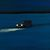# How to get the mid point (X,Y) of a polyline segment in Arcade ?

2722
10
09-09-2020 05:21 AMby Anonymous User
Not applicable

I have a road layer with many segmented lines, I want to create a pop up link using the mid point x,y info.

Thank you so much !!

Tags (4)
1 Solution

Accepted SolutionsbyEsri Esteemed Contributor

Hi KhattabK ,

Not entirely sure if this works, but could you try it?

``````Function dist(x1, y1, x2, y2) {
return Sqrt(Pow(x2-x1, 2) + Pow(y2-y1, 2));
}

// get the geometry
var segment = Geometry(\$feature)["paths"];
// determine length halfway
var halflen = Length(\$feature) / 2;

// initialize vars
var xcrd = Null;
var ycrd = Null;
var cumlen = 0;

// loop through coordinate pairs in segment
for (var i in segment) {
if (i > 0) {
// second coordinate pair, start calculating distance
var xprev = segment[i-1]["x"];
var yprev = segment[i-1]["y"];
var xcurr = segment[i]["x"];
var ycurr = segment[i]["y"];
var len = dist(xprev, yprev, xcurr, ycurr);
var prevcum = cumlen;
cumlen += len;
// if past halfway on line
if (cumlen > halflen) {
// calculate midpoint
var restlen = halflen - prevcum;
var frac = restlen / len;
var xmid = (xcurr - xprev) * frac + xprev;
var ymid = (ycurr - yprev) * frac + yprev;
return "(" + Round(xmid, 2) + ", " + Round(ymid, 2) + ")";
}
} else {
// first coordinate pair
var xcrd = segment[i]["x"];
var ycrd = segment[i]["y"];
}
}

return "Error";
‍‍‍‍‍‍‍‍‍‍‍‍‍‍‍‍‍‍‍‍‍‍‍‍‍‍‍‍‍‍‍‍‍‍‍‍‍‍‍‍‍‍``````
10 RepliesbyEsri Esteemed Contributor

Hi KhattabK ,

Not entirely sure if this works, but could you try it?

``````Function dist(x1, y1, x2, y2) {
return Sqrt(Pow(x2-x1, 2) + Pow(y2-y1, 2));
}

// get the geometry
var segment = Geometry(\$feature)["paths"];
// determine length halfway
var halflen = Length(\$feature) / 2;

// initialize vars
var xcrd = Null;
var ycrd = Null;
var cumlen = 0;

// loop through coordinate pairs in segment
for (var i in segment) {
if (i > 0) {
// second coordinate pair, start calculating distance
var xprev = segment[i-1]["x"];
var yprev = segment[i-1]["y"];
var xcurr = segment[i]["x"];
var ycurr = segment[i]["y"];
var len = dist(xprev, yprev, xcurr, ycurr);
var prevcum = cumlen;
cumlen += len;
// if past halfway on line
if (cumlen > halflen) {
// calculate midpoint
var restlen = halflen - prevcum;
var frac = restlen / len;
var xmid = (xcurr - xprev) * frac + xprev;
var ymid = (ycurr - yprev) * frac + yprev;
return "(" + Round(xmid, 2) + ", " + Round(ymid, 2) + ")";
}
} else {
// first coordinate pair
var xcrd = segment[i]["x"];
var ycrd = segment[i]["y"];
}
}

return "Error";
‍‍‍‍‍‍‍‍‍‍‍‍‍‍‍‍‍‍‍‍‍‍‍‍‍‍‍‍‍‍‍‍‍‍‍‍‍‍‍‍‍‍``````by Anonymous User
Not applicable

Thank you so much for getting back to me so quickly ! I really appreciate your help.

This worked like a champ ! I had to add the Meters to Lat Long Function & concatenation to get what I wanted. I will share the source link here for the benefit. Lat/Long unit conversion with Arcade

I was wondering if you know if this is possible in Arcade :

The ability to grab the x,y of the user's mouse click and put it in Arcade for String Concatenation.   (please see snip below)

Thank you so much  Xander Bakker!!!!!byEsri Esteemed Contributor

Hi KhattabK ,

Unfortunately, it is not possible to access the exact location where the user clicked. It would be great to be able to do this. Currently for a pop-up you will have access (initially) to the feature geometry where the user clicked, but not the exact location. No problem for point data, but it will be for polylines and polygons.by Anonymous User
Not applicable

Thank you so much for the clarification!!New Contributor

As I am a novice with Arcade, I am loving this post.  Xander Bakker's post works great, but I am like you and need the Lat/Long.  You have the code link for that, but I am not sure how to add it to the original function.  Can you give me some advice as to where to insert it?Occasional Contributor III

Are you a wizard?by
Frequent Contributor

If for some reason that midpoint expression doesn't work, this might be an option:

Buffer the line and get the centroid of the resulting polygon. It's kind of like a "poor man's midpoint".

If I recall, that works ok. But I think I remember the point not being the exact midpoint of the line. I forget what the specific issue was. It's probably good enough for most use cases though...by Anonymous User
Not applicable

In Desktop in Toolbox you can use the Data Management > Features > Add Geometry Attributes.

Add the line you're wanting to determine the midpoint for as the Input Feature and check the box next to Line_Start_Mid_End.  This will add columns in the line's attribute table showing the coordinates for the Start X and Y, Mid X and Y, End X and Y in the same coordinate system as the line.  It doesn't actually create a point but you could then create a feature using these coordinates.by
Frequent Contributor

That's an interesting idea.

If I understand correctly, I think you're saying we could:

...reference those "Geometry Attributes" (fields in the attribute table but not in the underlying db table) right in Arcade. I think I've done the same sort of thing with the  \$feature.shape_length:  https://community.esri.com/t5/arcgis-pro-ideas/hascurve-arcade-geometry-property/idc-p/1149449/highl...

Is that what you were thinking?

Never mind. Those "Geometry Attribute" fields aren't dynamic.